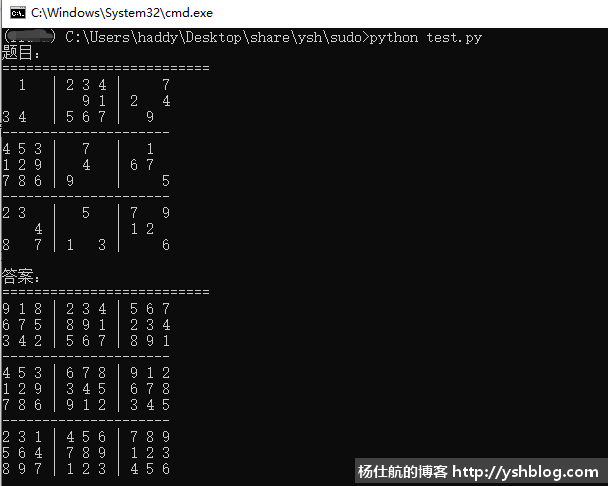### Python生成数独题目

• 发布时间：2020年3月12日 00:52
• 作者：杨仕航
• 分类标签： Python
• 阅读(19702)
• 评论(0)

### 1、初次尝试1）同一行的数字不能重复

2）同一列的数字不能重复

3）同一个九宫格的数字不能重复

1）先定义获取一个二维矩阵行、列、九宫格的方法

```import random
import numpy as np

# 其中sudo参数是numpy的二维矩阵
# 获取格子所在的行的全部格子
def get_row(sudo, row):
return sudo[row, :]

# 获取格子所在的列的全部格子
def get_col(sudo, col):
return sudo[:, col]

# 获取格子所在的九宫格的全部格子
def get_block(sudo, row, col):
row_start = row // 3 * 3
col_start = col // 3 * 3
return sudo[row_start: row_start + 3, col_start: col_start + 3]```

2）书写生成逻辑：遍历，排除已被使用的数字，随机填入

```def create():
# 1~9，参考一维数组
sample = np.array(range(1, 10))
# 9*9的二维矩阵，每个格子默认值为0
sudo = np.zeros((9, 9), dtype=int)

# 遍历从左到右，从上到下逐个遍历
for row in range(9):
for col in range(9):
# 获取该格子对应的行、列、九宫格
sudo_row = get_row(sudo, row)
sudo_col = get_col(sudo, col)
sudo_block = get_block(sudo, row, col)

# 该格子已被使用的数字，并集运算
used_nums = np.union1d(np.union1d(sudo_row, sudo_col), sudo_block)
# 剩下可用的数字,差集运算
rest_nums = np.setdiff1d(sample, used_nums)

# 判断是否有数字可用
if len(rest_nums) == 0:
raise Exception('发生错误，没有数字可用')

# 随机取出一个
rand_num = random.sample(rest_nums.tolist(),1)
sudo[row, col] = rand_num
return sudo```

3）执行```# 打印数独
def print_sudo(sudo):
for row_index, row in enumerate(sudo):
if row_index % 3 == 0 and row_index != 0:
print('-' * (9 + 8 + 4))
row = list(map(str, row.tolist()))
row.insert(6, '|')
row.insert(3, '|')
print(' '.join(row))

def create():
# 1~9，参考一维数组
sample = np.array(range(1, 10))
# 9*9的二维矩阵，每个格子默认值为0
sudo = np.zeros((9, 9), dtype=int)

# 遍历从左到右，从上到下逐个遍历
for row in range(9):
for col in range(9):
# 获取该格子对应的行、列、九宫格
sudo_row = get_row(sudo, row)
sudo_col = get_col(sudo, col)
sudo_block = get_block(sudo, row, col)

# 该格子已被使用的数字，并集运算
used_nums = np.union1d(np.union1d(sudo_row, sudo_col), sudo_block)
# 剩下可用的数字,差集运算
rest_nums = np.setdiff1d(sample, used_nums)

# 判断是否有数字可用
if len(rest_nums) == 0:
print_sudo(sudo)
raise Exception('发生错误，[{0}, {1}]没有数字可用'.format(row + 1, col + 1))

# 随机取出一个
rand_num = random.sample(rest_nums.tolist(),1)
sudo[row, col] = rand_num
return sudo```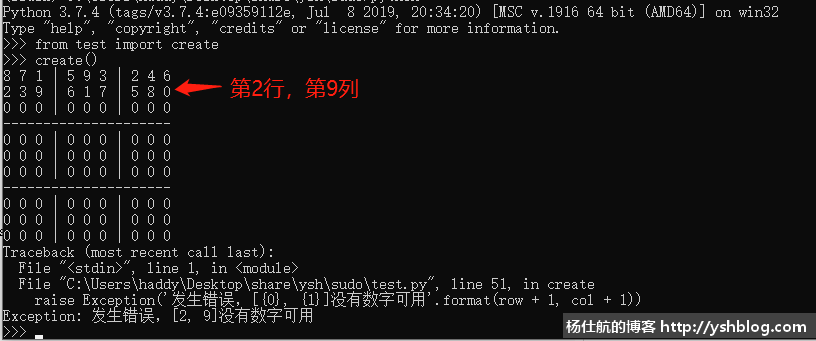4）初步结论

### 2、柳暗花明又一村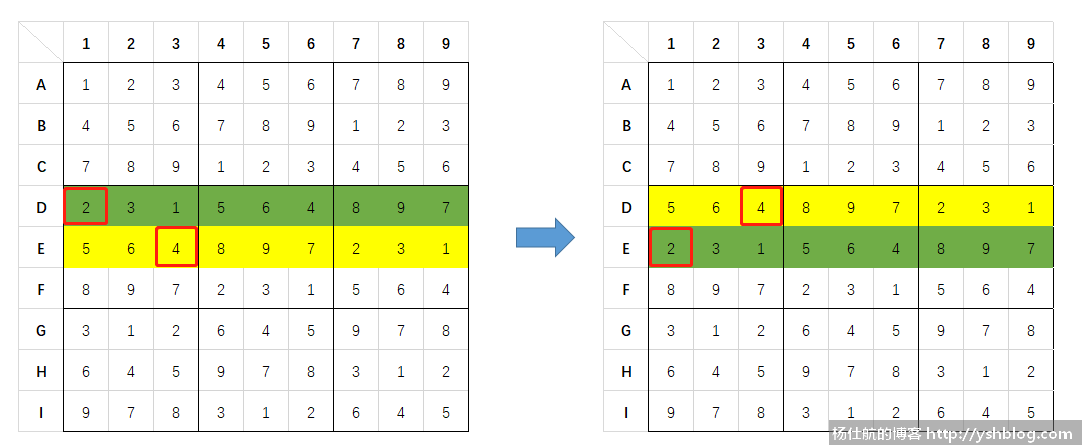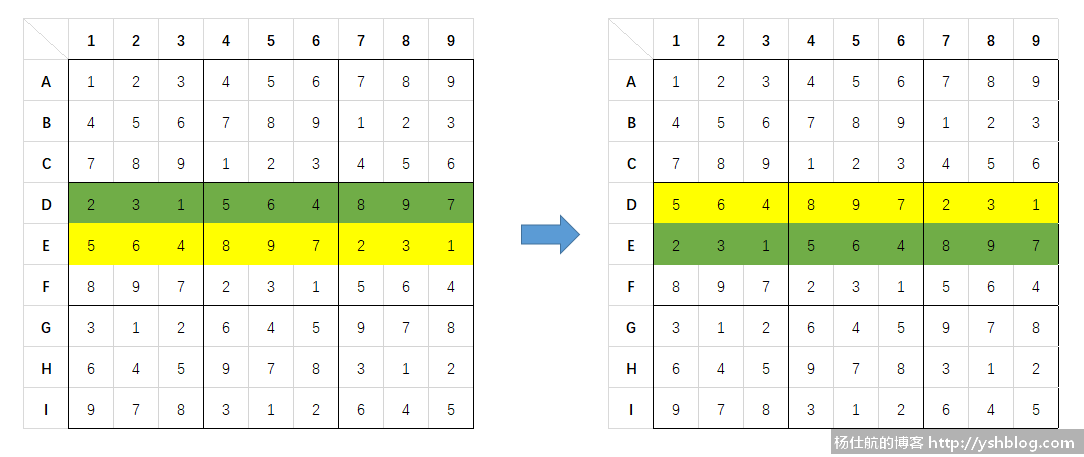1）生成基本盘

```def create_base_sudo():
# 9*9的二维矩阵，每个格子默认值为0
sudo = np.zeros((9, 9), dtype=int)
# 随机生成起始的基数(1 ~ 9)
num = random.randrange(9) + 1

# 遍历从左到右，从上到下逐个遍历
for row_index in range(9):
for col_index in range(9):
# 获取该格子对应的行、列、九宫格
sudo_row = get_row(sudo, row_index)
sudo_col = get_col(sudo, col_index)
sudo_block = get_block(sudo, row_index, col_index)

# 如果该数字已经存在于对应的行、列、九宫格
# 则继续判断下一个候选数字，直到没有重复
while num in sudo_row or num in sudo_col or num in sudo_block:
num = num % 9 + 1

# 赋值
sudo[row_index, col_index] = num
num = num % 9 + 1
return sudo```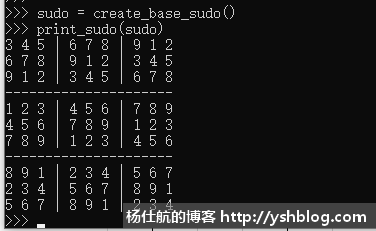2）随机交换，得到终盘

```# 随机交换n次
def random_sudo(sudo, times):
for _ in range(times):
# 随机交换两行
rand_row_base = random.randrange(3) * 3  # 从0，3，6 随机取一个
rand_rows = random.sample(range(3), 2)   # 从 0，1，2中随机取两个数
row_1 = rand_row_base + rand_rows
row_2 = rand_row_base + rand_rows
sudo[[row_1, row_2], :] = sudo[[row_2, row_1], :]

# 随机交换两列
rand_col_base = random.randrange(3) * 3
rand_cols = random.sample(range(3), 2)
col_1 = rand_col_base + rand_cols
col_2 = rand_col_base + rand_cols
sudo[:, [col_1, col_2]] = sudo[:, [col_2, col_1]]```3）随机抹去数字，得到数独题目

```def get_sudo_subject(sudo, del_nums):
# 最少要保留17个数字，题目才可解。一共81个数字，则最多可以擦除81-17 = 64。
max_clear_count = 64
# 最少也要擦除14个
min_clear_count = 14

if del_nums < min_clear_count:
del_nums = min_clear_count
if del_nums > max_clear_count:
del_nums = max_clear_count

# 随机擦除（从0到80，随机取要删除的个数）
clears = random.sample(range(81), del_nums)
for clear_index in clears:
# 把0到80的坐标转化成行和列索引
# 这样就不会重复删除同一个格子的数字
row_index = clear_index // 9
col_index = clear_index % 9
sudo[row_index, col_index] = 0```4）整合代码

```import random
import numpy as np

# 获取格子所在的行的全部格子
def get_row(sudo, row):
return sudo[row, :]

# 获取格子所在的列的全部格子
def get_col(sudo, col):
return sudo[:, col]

# 获取格子所在的九宫格的全部格子
def get_block(sudo, row, col):
row_start = row // 3 * 3
col_start = col // 3 * 3
return sudo[row_start: row_start + 3, col_start: col_start + 3]

# 打印数独
def print_sudo(sudo):
for row_index, row in enumerate(sudo):
if row_index % 3 == 0 and row_index != 0:
print('-' * (9 + 8 + 4))
row = list(map(str, row.tolist()))
row.insert(6, '|')
row.insert(3, '|')
row_str = ' '.join(row)
print(row_str.replace('0', ' '))

def create_base_sudo():
# 9*9的二维矩阵，每个格子默认值为0
sudo = np.zeros((9, 9), dtype=int)
# 随机生成起始的基数(1 ~ 9)
num = random.randrange(9) + 1

# 遍历从左到右，从上到下逐个遍历
for row_index in range(9):
for col_index in range(9):
# 获取该格子对应的行、列、九宫格
sudo_row = get_row(sudo, row_index)
sudo_col = get_col(sudo, col_index)
sudo_block = get_block(sudo, row_index, col_index)

# 如果该数字已经存在于对应的行、列、九宫格
# 则继续判断下一个候选数字，直到没有重复
while num in sudo_row or num in sudo_col or num in sudo_block:
num = num % 9 + 1

# 赋值
sudo[row_index, col_index] = num
num = num % 9 + 1
return sudo

def random_sudo(sudo, times):
for _ in range(times):
# 随机交换两行
rand_row_base = random.randrange(3) * 3  # 从0，3，6 随机取一个
rand_rows = random.sample(range(3), 2)   # 从 0，1，2中随机取两个数
row_1 = rand_row_base + rand_rows
row_2 = rand_row_base + rand_rows
sudo[[row_1, row_2], :] = sudo[[row_2, row_1], :]

# 随机交换两列
rand_col_base = random.randrange(3) * 3
rand_cols = random.sample(range(3), 2)
col_1 = rand_col_base + rand_cols
col_2 = rand_col_base + rand_cols
sudo[:, [col_1, col_2]] = sudo[:, [col_2, col_1]]

def get_sudo_subject(sudo, del_nums):
subject = sudo.copy()

# 随机擦除（从0到80，随机取要删除的个数）
clears = random.sample(range(81), del_nums)
for clear_index in clears:
# 把0到80的坐标转化成行和列索引
# 这样就不会重复删除同一个格子的数字
row_index = clear_index // 9
col_index = clear_index % 9
subject[row_index, col_index] = 0
return subject

if __name__ == '__main__':
max_clear_count = 64  # 最多清除个数
min_clear_count = 14  # 最少清除个数

# 设置难度等级，1~5，5个等级：入门、初级、熟练、精通、大神
level = 3

# 每个等级的个数
each_level_count = (max_clear_count - min_clear_count) / 5

# 该等级最小数
level_start = min_clear_count + (level - 1) * each_level_count
# 该等级范围内的随机数
del_nums = random.randrange(level_start, level_start + each_level_count)

# 生成基本盘
sudo = create_base_sudo()
# 生成终盘
random_sudo(sudo, 50)
# 获取数独题目
subject = get_sudo_subject(sudo, del_nums)

print('题目：')
print('=' * 26)
print_sudo(subject)
print('\n答案：')
print('=' * 26)
print_sudo(sudo)```